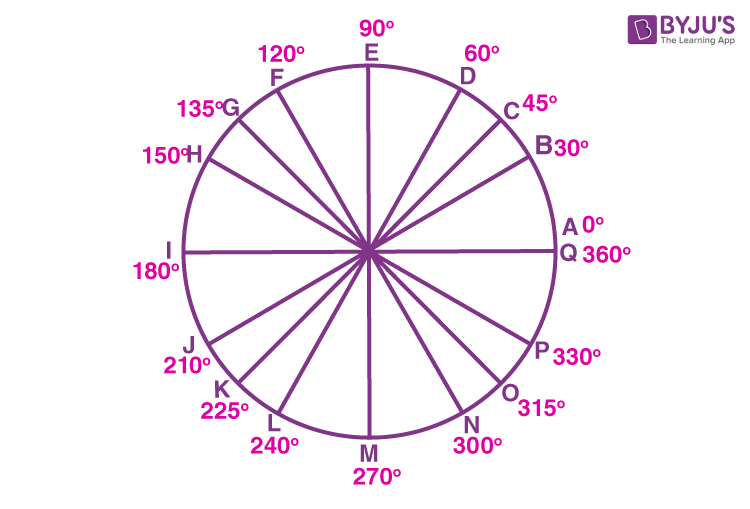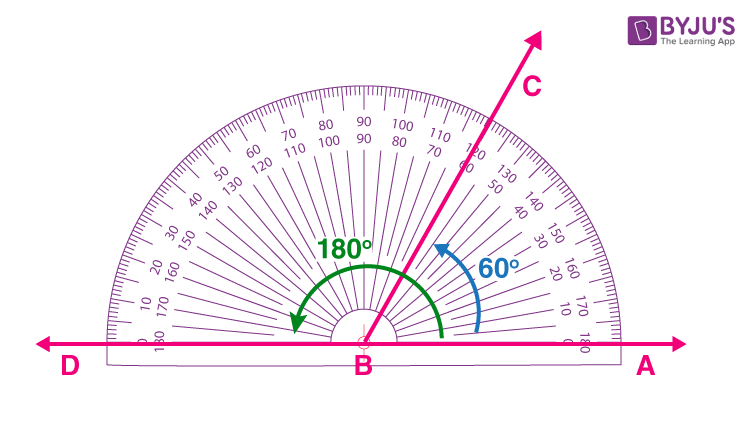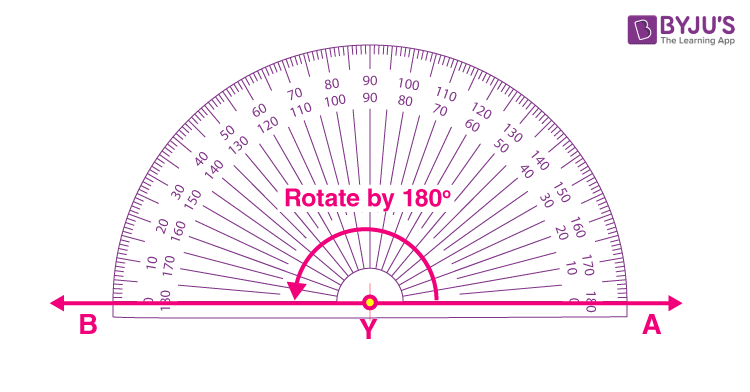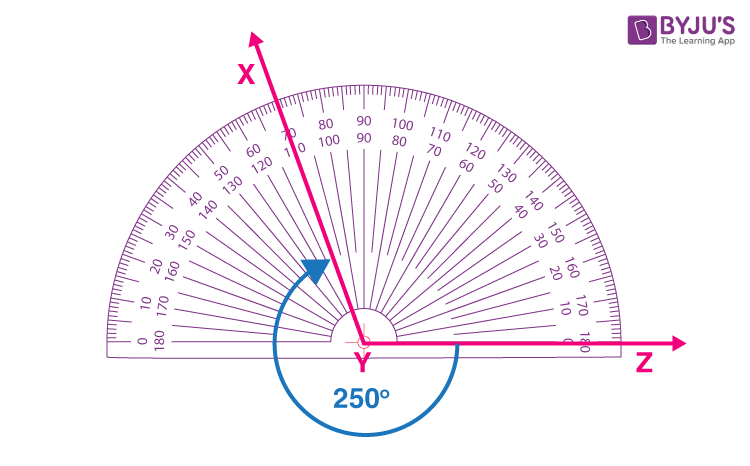# Degree Measure

In geometry, the measure of rotation of a given ray about its initial point is called angle. The primary ray is called the initial side, and the final position of the ray after rotation is called the terminal side of the angle. The point of rotation is called the vertex. The angle is positive if the direction of rotation is anticlockwise, whereas the angle is negative if the direction of rotation is clockwise. The measure of an angle is the amount of rotation made to get the terminal side from the initial side. There are many units for measuring angles, such as degree measure and radian measure. In this article, you will learn what degree measurement is and how to get the degree measurement of an angle with examples.

## Degree Measure of an Angle

The degree measure is represented using “°” and is called a degree symbol. The measure of an angle is said to be one degree, i.e. 1°, if the rotation from the initial side to the terminal side is (1/360)th of a revolution as one complete revolution means the initial and terminal sides coincide. In other words, an angle equal to 360 degrees is called full rotation or full angle. It is formed when one of the arms makes a complete rotation to form an angle. The below figure shows the degree measure of an angle at different positions.Here, OA is the initial side and OB, OC, OD, OE, OF, OG, OH, OI, OJ, OK, OL, OM, ON, OP, OQ are terminal sides each of which measure certain angles. However, the initial side OA and terminal side OQ coincide with each other after one complete revolution. Thus, it made an angle of 360 degrees.

### Degree Measure Formula

Some of the important facts about the degree measure are:

• A degree is divided into 60 minutes, and a minute is divided into 60 seconds.
• One sixtieth of a degree is called a minute, written as 1′.
• One sixtieth of a minute is called a second, written as 1″.

The above statements can be written as:

1° = 60′ (one degree = 60 minutes)

1′ = 60″ (one minute = 60 seconds)

(1/60)° = 1′ (One sixtieth of a degree = One minute)

(1/60)′ = 1″ (One sixtieth of a minute = One second)

### Degree Measure Tool

The protractor is one the geometric tools, used to measure the degree of an angle. The below points should be remembered while measuring an angle using the degree measurement tool, i.e. protractor.

• If the angle is to be measured from the left side of the protractor, we have to consider the outer reading provided on the protractor.
• If the angle is measured from the protractor’s right side, we have to consider the inner reading provided on the protractor.

Some of the angles and their measurements using protractor are given below to understand the concept in a better way.

Example 1: Measure the angle 60 degrees.

In the below figure, the angle is measured in degrees from the right side of the protractor. Thus, ∠ABC = 60 °If we measure the angle from the left side of the protractor, then ∠CBD = 120°. As we can notice that ∠ABC and ∠CBD have the same terminal side and their initial sides are the segments of the ray AB. The angle measure is different from one another because of the way we consider the reading.

Example 2: What is The degree Measure of a Straight Angle

The degree measure of a straight angle is 180 degrees, This can be shown as:Example 3: Show the 250 degrees measure of angle.

Let us write the angle 250 degrees as the sum of two angles so that we can easily mark the angle using a protractor.

250° = 180° + 70°

So, first we need to rotate the protractor for 180 degrees and then measure 70 degrees. This is given in the figure below.Similarly, we can find the degree measure of an arc and central angle. Also, learn the measurement of angles with examples here.

### Degree Measure of 1 Radian

Another unit for the measurement of an angle is the radian measure. However, both degree and radian measures can be converted from one unit to another. The degree measure of 1 radian is given by:

1 radian = 180°/π = 57°16′ (approx)

The above relation is used to convert the measurement of angles from degrees to radians and vice versa.